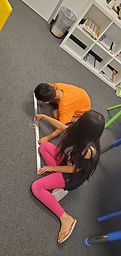## Mr. Jake

### Target 1​

###### Lesson Type:

New

Number Operation

:

Computation

Solve multi-step word problems within 1,000,000 (addition, subtraction, multiplication, and division).

###### 1:

Identify the correct arithmetic processes based on the information presented in word problems.

###### 2:

Find the key words in word problems that indicate the correct arithmetic process to use: Addition: add, altogether, both, combined, in all, plus, sum, total; Subtraction: difference, fewer, how many/much more, left, less, minus, remains; Multiplication: times, product, each, twice; and Division: how many in each, divided by, quotient, goes into, split evenly.

###### 3:

Find and use the needed information in a word problem to solve.

6th

###### Vocabulary:

Variable, Arithmetic

Activities:

Identified the important information in word problems

Used inverse operations to solve word problems with variables

Practiced creating their own word problems### Home Exploration

###### Guiding Questions:## Absent Students:

### Target 2

:

###### 1:

Understand circumference is the distance around the edge of a circle.

###### 2:

Identify the center of a circle and measure the radius.

###### 3:

Measure the diameter of given circles.

###### 4:

Recognize that a circle has many different radii and diameters passing through its center.

###### 5:

Understand the relationship between the radius and the diameter of a circle (use the radius to find the diameter or the diameter to find the radius).

7th

###### Vocabulary:

Activities:

Measured radius, diameter, and circumference of circles

Discussed what they know about circles and circle measurements### Home Exploration

###### Guiding Questions:### Target 3

:

###### Vocabulary:

Activities:### Home Exploration